JEE  >  Test: 35 Year JEE Previous Year Questions: Thermodynamics

# Test: 35 Year JEE Previous Year Questions: Thermodynamics

Test Description

## 31 Questions MCQ Test Chemistry for JEE | Test: 35 Year JEE Previous Year Questions: Thermodynamics

Test: 35 Year JEE Previous Year Questions: Thermodynamics for JEE 2022 is part of Chemistry for JEE preparation. The Test: 35 Year JEE Previous Year Questions: Thermodynamics questions and answers have been prepared according to the JEE exam syllabus.The Test: 35 Year JEE Previous Year Questions: Thermodynamics MCQs are made for JEE 2022 Exam. Find important definitions, questions, notes, meanings, examples, exercises, MCQs and online tests for Test: 35 Year JEE Previous Year Questions: Thermodynamics below.
Solutions of Test: 35 Year JEE Previous Year Questions: Thermodynamics questions in English are available as part of our Chemistry for JEE for JEE & Test: 35 Year JEE Previous Year Questions: Thermodynamics solutions in Hindi for Chemistry for JEE course. Download more important topics, notes, lectures and mock test series for JEE Exam by signing up for free. Attempt Test: 35 Year JEE Previous Year Questions: Thermodynamics | 31 questions in 60 minutes | Mock test for JEE preparation | Free important questions MCQ to study Chemistry for JEE for JEE Exam | Download free PDF with solutions
 1 Crore+ students have signed up on EduRev. Have you?
Test: 35 Year JEE Previous Year Questions: Thermodynamics - Question 1

### If an endothermic reaction is non-spontaneous at freezing point of water and becomes feasible at its boiling point, then

Detailed Solution for Test: 35 Year JEE Previous Year Questions: Thermodynamics - Question 1

TIPS/Formulae : ΔG = ΔH – TΔS
Since ΔG = ΔH – TΔS for an endothermic reaction, ΔH = +ve and at low temperature ΔS = + ve
Hence ΔG = (+) ΔH – T( + )ΔS and if TΔS < ΔH (at low temp) ΔG  = +ve (non spon taneous) But at high temperature, reaction becomes spontaneous i.e. ΔG = –ve. because at higher temperature TΔS > ΔH.

Test: 35 Year JEE Previous Year Questions: Thermodynamics - Question 2

### A heat engine abosrbs heat Q1 at temperature T1 and heat Q2 at temperature T2. Work done by the engine is J (Q1 + Q2). This data 

Detailed Solution for Test: 35 Year JEE Previous Year Questions: Thermodynamics - Question 2

According to first law of thermodynamics energy can neither be created nor destroyed although it can be converted from one form to another.
NOTE :  Carnot cycle is based upon this principle but ΔUring the conversion of heat into work some mechanical energy is always converted to other form of energy hence this data violates 1st law of thermodynamics.

Test: 35 Year JEE Previous Year Questions: Thermodynamics - Question 3

### For the reactions,  2C + O2 → 2CO2 ; ΔH = -393 J 2Zn + O2 → 2ZnO ; ΔH = -412 J

Detailed Solution for Test: 35 Year JEE Previous Year Questions: Thermodynamics - Question 3

ΔH negative shows that the reaction is spontaneous.
Higher negative value for Zn shows that the reaction is more feasible.

Test: 35 Year JEE Previous Year Questions: Thermodynamics - Question 4

The heat required to raise the temperature of body by 1 K is called 

Detailed Solution for Test: 35 Year JEE Previous Year Questions: Thermodynamics - Question 4

The heat required to raise the temperature of body by 1K is called thermal capacity or heat capacity.

Test: 35 Year JEE Previous Year Questions: Thermodynamics - Question 5

The internal energy change when a system goes from state A to B is 40 kJ/mole. If the system goes from A to B by a reversible path and returns to state A by an irreversible path what would be the net change in internal energy ? 

Detailed Solution for Test: 35 Year JEE Previous Year Questions: Thermodynamics - Question 5

For a cyclic process then net change in the internal energy is zero because the change in internal energy does not depend on the path.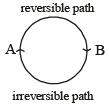Test: 35 Year JEE Previous Year Questions: Thermodynamics - Question 6

If at 298 K the bond energies of C — H, C — C, C = C and H — H bonds are respectively 414, 347, 615 and 435 kJ mol–1, the value of enthalpy change for the reaction H2C = CH2(g) + H2(g) → H3C — CH3(g) at 298 K will be 

Detailed Solution for Test: 35 Year JEE Previous Year Questions: Thermodynamics - Question 6

CH2 = CH2(g) + H2(g) → CH3 - CH3
Enthalpy change = Bond energy of reactants – Bond energy of products.
ΔH = 1(C = C) + 4 (C – H) + 1 (H - H) - 1 (C - C) - 6 (C - H)
= 1 (C = C) + 1 ( H – H) – 1 (C – C) – 2 (C– H)
= 615 + 435 – 347 – 2 × 414  = 1050 – 1175 = –125 kJ.

Test: 35 Year JEE Previous Year Questions: Thermodynamics - Question 7

In an irreversible process taking place at constant T and P and in which only pressure-volume work is being done, the change in Gibbs free energy (ΔG) and  change in entropy (dS), satisfy the criteria 

Detailed Solution for Test: 35 Year JEE Previous Year Questions: Thermodynamics - Question 7

For spontaneous reaction, dS > 0 and ΔG should be negative i.e. < 0.

Test: 35 Year JEE Previous Year Questions: Thermodynamics - Question 8

The correct relationship between free energy change in a reaction and the corresponding equilibrium constant Kc is 

Detailed Solution for Test: 35 Year JEE Previous Year Questions: Thermodynamics - Question 8

ΔGº = – RT lnKc or – ΔGº = RT lnKc

Test: 35 Year JEE Previous Year Questions: Thermodynamics - Question 9

The enthalpy change for a reaction does not depend upon 

Detailed Solution for Test: 35 Year JEE Previous Year Questions: Thermodynamics - Question 9

Enthalpy change for a reaction does not depend upon the nature of intermediate reaction steps.

Test: 35 Year JEE Previous Year Questions: Thermodynamics - Question 10

An ideal gas expands in volume from 1×10–3 to 1 × 10–2 m3 at 300 K against a constant pressure of 1×105 Nm–2. The work done is 

Detailed Solution for Test: 35 Year JEE Previous Year Questions: Thermodynamics - Question 10

w = -PΔV = -10-5 (1x10 -2 - 1x10 -3) = -900J

Test: 35 Year JEE Previous Year Questions: Thermodynamics - Question 11

The enthalpies of combustion of carbon and carbon monoxide are – 393.5 and – 283 kJ mol–1 respectively. The enthalpy of formation of carbon monoxide per mole is

Detailed Solution for Test: 35 Year JEE Previous Year Questions: Thermodynamics - Question 11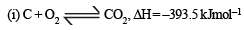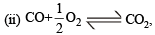ΔH = –283.0 kJmol–1

Operating (i) - (ii), we have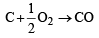ΔH = -110.5 kJmol–1

Test: 35 Year JEE Previous Year Questions: Thermodynamics - Question 12

Consider an endothermic reaction X → Y with the activation en er gies Eb and Ef  for the backward and forward reactions, respectively. In general 

Detailed Solution for Test: 35 Year JEE Previous Year Questions: Thermodynamics - Question 12

Enthalpy of reaction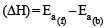for an endothermic reaction ΔH = +ve hence for ΔH to be negative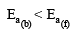Test: 35 Year JEE Previous Year Questions: Thermodynamics - Question 13

Consider the reaction : N2 + 3H2 → 2 NH3 carried out at constant temperature and pressure. If ΔH and ΔU are the enthalpy and internal energy changes for the reaction, which of the following expressions is true ? 

Detailed Solution for Test: 35 Year JEE Previous Year Questions: Thermodynamics - Question 13

ΔH = ΔU + ΔnRT for N2 + 3H2 —​→ 2 NH3
Δng = 2 – 4 = – 2
∴ΔH =ΔU-2RT or ΔU = ΔH+2RT ∴ΔU >ΔH

Test: 35 Year JEE Previous Year Questions: Thermodynamics - Question 14

If the bond dissociation energies of XY, X 2 and Y2 (all diatomic molecules) are in the ratio of 1 : 1 : 0.5 and ΔHf for the for mation of XY is – 200 kJ mole -1 . The bond dissociation energy of X2 will be 

Detailed Solution for Test: 35 Year JEE Previous Year Questions: Thermodynamics - Question 14

X2+Y2→2XY

ΔH=(BE)XX+(BE)YY–2(BE)XY

If (BE) of XY = a then (BE) of (XX)=a

and (BE) of (YY)=a/2

So, ΔHf(XY)=−200kJ

So, −400 (for 2 moles XY)=a+a/2–2a

⇒−400=−a/2

a=800kJ

The bond dissociation energy of X2=800kJ/mol

Test: 35 Year JEE Previous Year Questions: Thermodynamics - Question 15

An ideal gas is allowed to expand both reversibly and irreversibly in an isolated system. If Ti is the initial temperature and Tf is the final temperature, which of the following statements is correct? 

Detailed Solution for Test: 35 Year JEE Previous Year Questions: Thermodynamics - Question 15

NOTE : In a reversible process the work done is greater than in irreversible process. Hence the heat absorbed in reversible process would be greater than in the latter case. So
Tf(rev.) < Tf (irr.)

Test: 35 Year JEE Previous Year Questions: Thermodynamics - Question 16

The standard enthalpy of formation (DfHº) at 298 K for methane, CH4(g) is –74.8 kJ mol–1. The additional information required to determine the average energy for C – H bond formation would be 

Detailed Solution for Test: 35 Year JEE Previous Year Questions: Thermodynamics - Question 16

The standard enthalpy of formation of CH4 is given by the equation : C(s) + 2H2(g) —​→ CH4(g)

Hence, dissociation energy of hydrogen and enthalpy of sublimation of carbon is required.

Test: 35 Year JEE Previous Year Questions: Thermodynamics - Question 17

The enthalpy changes for the following processes are listed below :      
Cl2(g) → 2Cl(g), 242.3 kJ mol–1
I2(g) → 2I(g), 151.0 kJ mol–1
ICl(g) → I(g) + Cl(g), 211.3 kJ mol–1I2(s) → I2(g), 62.76 kJ mol–1
Given that the standard states for iodine and chlorine are I2(s) and Cl2(g), the standard enthalpy of formation for ICl(g) is : 

Detailed Solution for Test: 35 Year JEE Previous Year Questions: Thermodynamics - Question 17

I2 (s) + Cl2(g) —→ 2ICl(g)
ΔA = [ΔI2(s) →I2(g) + ΔHI–I + ΔHCl–Cl] – 2[ΔHI – Cl]
=  151.0 + 242.3 + 62.76 –2 × 211.3 = 33.46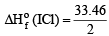= 16.73 kJ / mol

Test: 35 Year JEE Previous Year Questions: Thermodynamics - Question 18

(ΔH – ΔU) for the formation of carbon monoxide (CO) from its elements at 298 K is  (R = 8.314 J K–1 mol–1

Detailed Solution for Test: 35 Year JEE Previous Year Questions: Thermodynamics - Question 18

For the reaction,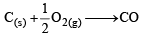ΔH = ΔU + DnRT  or ΔH – ΔU = DnRT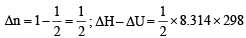= 1238.78 J mol–1

Test: 35 Year JEE Previous Year Questions: Thermodynamics - Question 19

In conversion of lime-stone to lime, CaCO3 (s) → CaO(s)+ CO2(g) the values of ΔH° and ΔS° are + 179.1 kJ mol-1 and 160.2 J/K respectively at 298 K and 1 bar. Assuming that ΔH° and ΔS° do not change with temperature, temperature above which conversion of limestone to lime will be spontaneous is 

Detailed Solution for Test: 35 Year JEE Previous Year Questions: Thermodynamics - Question 19

ΔG° = ΔH° – TΔS°

For a spontaneous reaction ΔG° < 0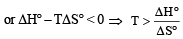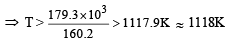Test: 35 Year JEE Previous Year Questions: Thermodynamics - Question 20

Assuming that water vapour is an ideal gas, the internal energy change (ΔU) when 1 mol of water is vapourised at 1 bar pressure and 100°C, (given : molar enthalpy of vapourisation of water at 1 bar and 373 K = 41 kJ mol–1 and R = 8.3 J mol–1 K–1) will be 

Detailed Solution for Test: 35 Year JEE Previous Year Questions: Thermodynamics - Question 20

Given ΔH = 41 kJ mol–1 = 41000 J mol–1
T = 100°C = 273 + 100 = 373 K, n = 1
ΔU = ΔH – DnRT = 41000 – (2 × 8.314 × 373)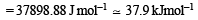Test: 35 Year JEE Previous Year Questions: Thermodynamics - Question 21

Identify the correct statement regarding a spontaneous process: 

Detailed Solution for Test: 35 Year JEE Previous Year Questions: Thermodynamics - Question 21

Spontaneity of reaction depen ds on tendency to acquire minimum energy state and maximum randomness. For a spontaneous process in an isolated system the change in entropy is positive.

Test: 35 Year JEE Previous Year Questions: Thermodynamics - Question 22

Oxidising power of chlorine in aqueous solution can be determined by the parameters indicated below: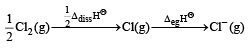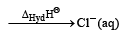(using the data,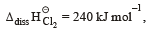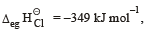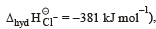will be 

Detailed Solution for Test: 35 Year JEE Previous Year Questions: Thermodynamics - Question 22

The energy involved in the conversion of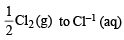is given by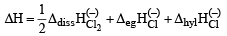Substituting various values from given data, we get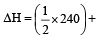(–349) (–381) kJmol–1

= (120 – 349 – 381) kJ mol–1 = – 610 kJ mol–1
i.e.,  the correct answer is (b)

Test: 35 Year JEE Previous Year Questions: Thermodynamics - Question 23

Standard entropy of X2, Y2 and X Y3 are 60, 40 and 50 J K–1 mol–1, respectively. For the reaction,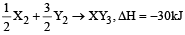, to be at equilibrium, the temperature will be 

Detailed Solution for Test: 35 Year JEE Previous Year Questions: Thermodynamics - Question 23

For a r eaction to be at equilibrium ΔG = 0.
Since ΔG = ΔH – TΔS so at equilibrium
ΔH – TΔS=0 or  ΔH = TΔS For the reaction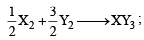ΔH= –30kJ (given)
Calculating ΔS for the above reaction, we get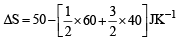=50 – (30 + 60) JK–1 = – 40 JK–1
At equilibrium,      TΔS =ΔH [∵ ΔG= 0]
∴ T x (–40) = –30x 1000 [∵ 1kJ = 1000J]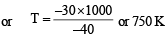Test: 35 Year JEE Previous Year Questions: Thermodynamics - Question 24

On the basis of the following thermochemical data :
f G°H+ ​(aq)= 0)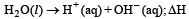= 57.32kJ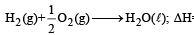= –286.20kJ

The value of enthalpy of formation of OH ion at 25° C is:

Detailed Solution for Test: 35 Year JEE Previous Year Questions: Thermodynamics - Question 24

Given, for reaction

(i)H2O (l) —​→ H+ (aq.) + OH (aq.); ΔHr= 57.32 kJ

(ii)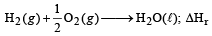= –286.20 kJ

For  reaction (i)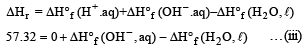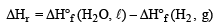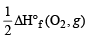–286.20 = ΔH°f (H2O, ℓ)

On replacing this value in equ. (iii) we have
57.32 =ΔH°f (OH– , aq) – (–286.20)
ΔH°f = –286.20+ 57.32 = –228.88 kJ

Test: 35 Year JEE Previous Year Questions: Thermodynamics - Question 25

The standard enthalpy of for mation of NH3 is – 46.0 kJ mol–1. If the enthalpy of formation of H2 from its atoms is – 436 kJ mol–1 and that of N2 is – 712 kJ mol–1, the average bond enthalpy of N – H bond in NH3 is 

Detailed Solution for Test: 35 Year JEE Previous Year Questions: Thermodynamics - Question 25

N2 + 3H2 —​→ 2 NH3 ΔH = 2 x-46.0 kJ mol–1
Let x be the bond enthalpy of N – H bond then
[Note : Enthalpy of formation or bond formation enthalpy is given which is negative but the given reaction involves bond breaking hence values should be taken as positive.]
ΔH = S Bond energies of products – S
Bond energies of reactants 2 × – 46 = 712 + 3 × (436) – 6x; – 92 = 2020 – 6x
6x = 2020 + 92 ⇒ 6x = 2112 ⇒  x = + 352 kJ/mol

Test: 35 Year JEE Previous Year Questions: Thermodynamics - Question 26

For a particular reversible reaction at temperature T, ΔH and ΔS were found to be both +ve. If Te is the temperature at equilibrium, the reaction would be spontaneous when 

Detailed Solution for Test: 35 Year JEE Previous Year Questions: Thermodynamics - Question 26

At equilibrium  ΔG = 0

Hence, ΔG = ΔH – TeΔS = 0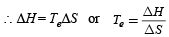For a spontaneous reaction
ΔG must be negative which is possible only if ΔH < TΔS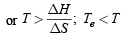Test: 35 Year JEE Previous Year Questions: Thermodynamics - Question 27

The entropy change involved in the isothermal reversible expansion of  2 mole of an ideal gas from a volume of 10 dm3 to a volume of 100 dm3 at 27°C is: 

Detailed Solution for Test: 35 Year JEE Previous Year Questions: Thermodynamics - Question 27

Entropy change for an isothermal reversible process is given by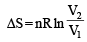= 2 × 8.314 × 2.303 log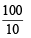= 38.3 J mol–1 K–1

Test: 35 Year JEE Previous Year Questions: Thermodynamics - Question 28

The incorrect expression among the following is : 

Detailed Solution for Test: 35 Year JEE Previous Year Questions: Thermodynamics - Question 28

ΔG° = ΔH° – TΔS°; – RT ℓnK = ΔH° – TΔS°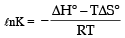Test: 35 Year JEE Previous Year Questions: Thermodynamics - Question 29

A piston filled with 0.04 mol of an ideal gas expands reversibly from 50.0 mL to 375 mL at a constant temperature of 37.0ºC. As it does so, it absorbs 208 J of heat. The values of q and w for the process will be: [JEE M 2013]
(R = 8.314 J/mol K) (ln 7.5 = 2.01)

Test: 35 Year JEE Previous Year Questions: Thermodynamics - Question 30

For complete combustion of ethanol, C2H5OH (l) + 3O2(g)—​→ 2CO2 ( g)+ 3H2O (l), the amount of heat produced as measured in bomb calorimeter, is 1364.47 kJ mol–1 at 25ºC.  Assuming ideality the enthalpy of combustion, ΔcH, for the reaction will be: (R = 8.314 kJ mol–1)         [JEE M 2014]

Detailed Solution for Test: 35 Year JEE Previous Year Questions: Thermodynamics - Question 30

C2 H5OH(l) + 3O2( g)→ 2CO2( g ) + 3H2O(l)

Bomb calorimeter gives ΔU of the reaction

Given, ΔU = –1364.47 kJ mol–1

Δng = – 1 ΔH = ΔU + ΔngRT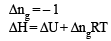= 1364.47 -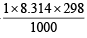= – 1366.93 kJ mol–1

Test: 35 Year JEE Previous Year Questions: Thermodynamics - Question 31

The heats of combustion of carbon an d car bon monoxide are –393.5 and –283.5 kJ mol–1, respectively. The heat of formation (in kJ) of carbon monoxide per mole is :          [JEE M 2016]

Detailed Solution for Test: 35 Year JEE Previous Year Questions: Thermodynamics - Question 31

Given C(s) + O2(g) → CO2(g); ΔH = –393.5 kJ mol–1 …(i)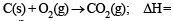= –393.5 kJ mol–1…(i)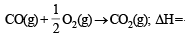–283.5 kJ mol–1 …(ii)

∴ Heat of formation of CO = eqn(i) – eqn(ii)

= –393.5 – (–283.5) = –110 kJ

## Chemistry for JEE

322 videos|586 docs|368 tests
 Use Code STAYHOME200 and get INR 200 additional OFF Use Coupon Code
Information about Test: 35 Year JEE Previous Year Questions: Thermodynamics Page
In this test you can find the Exam questions for Test: 35 Year JEE Previous Year Questions: Thermodynamics solved & explained in the simplest way possible. Besides giving Questions and answers for Test: 35 Year JEE Previous Year Questions: Thermodynamics, EduRev gives you an ample number of Online tests for practice

## Chemistry for JEE

322 videos|586 docs|368 tests